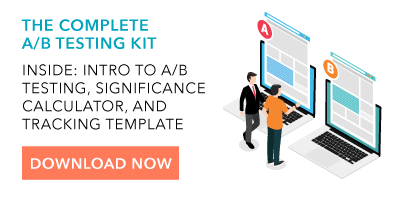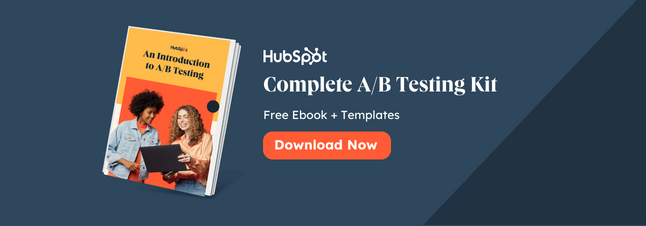What are some of the reasons you run an A/B test?

When I think of the benefits of A/B testing, I think of one of the most popular and concrete ways to experiment with ad designs that are effective for target audiences. I think of how changing one simple element can be the deciding factor for customers, and that running a test will help me figure out the preferred design.

Up until recently, I thought that there was only one kind of A/B test. After all, the definition itself is pretty straightforward.Then, I came across a different kind of A/B test. This method still involves testing variants to discover the preference of an audience, but it involves more calculation, and more trial and error.

This method is called Bayesian A/B testing, and if you want to take a more specific, tactical approach to your ad testing, this might be the answer.

But first, let's talk about how Bayesian A/B testing is different from traditional A/B tests.

### Bayesian A/B Testing

There are two types of A/B tests: Frequentist and Bayesian.

Every A/B test has the same few components. They use data, based on a metric, that determines variants A and B. For example, a metric can be the amount of times an ad is clicked. To determine the winner, that metric is measured statistically.

Let's apply this to an example of using the frequentist, or traditional, approach. In this scenario, you would design two ads and change one variable, such as the copy of the ad. Then, pick the metric, like the amount of times an ad is clicked.

The winner of the frequentist A/B test in this example would be which ad was clicked the most by your target audience based solely on results from that experiment.

If you were to illustrate these components in a Bayesian A/B test, you would approach the test using different data.

That definition can sound a little difficult to visualize without an example, so let's go over one.

If your previous ad on Facebook drew 867 unique visitors and acquired 360 conversions, earning a 41% conversion rate, you would use that data to inform an expectation. If you were to figure that your next Facebook ad reached 5,000 unique visitors, you could infer that you'd earn 2,050 conversions based on that prior experience. This would be variant "A."

Let's say you look at a similar Facebook ad's performance and ultimately earned a 52% conversion rate. This is variant "B." What you have done by collecting the data from the two variants is to calculate the posterior distribution, and the previous tests you’ve run have now become the ground for your Bayesian test.

If, before calculating the posterior distribution, you had inferences about conversion rates earned from each variable, you can now update them based on the data you've collected. You can ask hypothetical questions about your test, such as "How likely is it that 'B' will be larger than variant 'A'?" In this case, you can infer that the answer to this is 9%.

Then, the trial and error portion begins.

Bayesian methodology makes decisions by doing some inference. You can calculate expected loss by the rate your metric decreases when choosing either variable. Set a boundary, such as 2%, that the metric should drop below. Once you have collected enough data to support that a variant dropped below 2%, you'll have your test winner.

Because your inferred loss for a variant is the average amount of what your metric would decrease by if you chose said variant, your boundary should be small enough to comfortably suggest making a mistake that large.

The methodology suggests that you are more willing to make a mistake of a certain amount, then move on to a more refined experiment instead of wasting time on a mistake that dropped below that threshold.

If you were to run two experiments, they would stop when the expected loss is below that 4% boundary. You would use the values of your variants to calculate your average loss. Then, you would begin the test again using these values as your prosperity distribution.

Bayesian A/B testing proves that you can make a business decision that won't fall below that boundary you set. You can use the data you've collected to continuously run tests until you see metrics increase with each experiment.

When you use Bayesian testing, you can modify the test periodically and improve the results as the test runs. Bayesian A/B testing uses constant innovation to give you concrete results by making small improvements in increments. You don't have to use inference as a result, but instead, use it as a variant.

If you're running A/B tests on software or different channels, you don't have to change them to run a Bayesian A/B test. Instead, you can look at the tools you have at your disposal in that software to give you more calculated results. Then, you can continuously run those tests and analyze them to pick your winners.

You might use a Bayesian A/B test instead of a traditional A/B test if you want to factor in more metrics into your findings. This is a really good test to calculate a more concrete ROI on ads. Of course, if you have less time on your hands, you can always use a frequentist approach to get more of a "big picture" conclusion.

Whichever method you choose, A/B testing is popular because it gives you an inference that can be useful for you in future campaigns.Originally published Jun 5, 2020 4:00:00 AM, updated June 05 2020

Topics:

A/B Testing